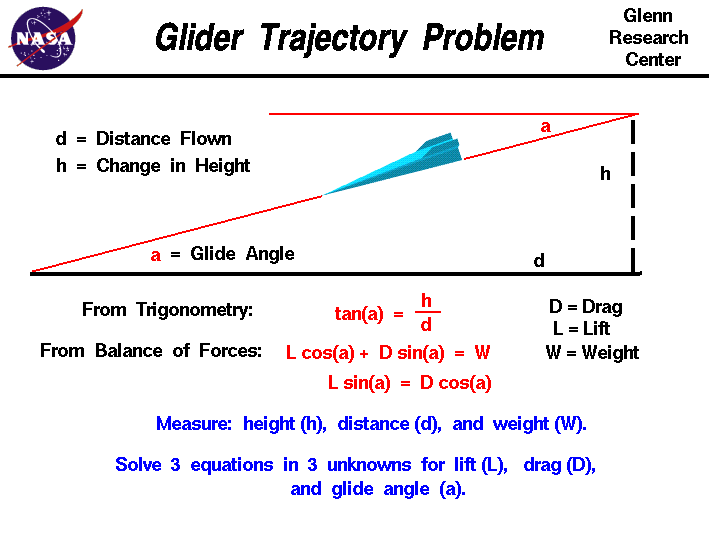+ Text Only Site
+ Non-Flash Version
+ Contact GlennDisplacement Activity If so instructed by your teacher, print out a worksheet page for these problems. More on the GliderYou will explore the trigonometric relationship tan (a) = h/d where a is the glide angle, h is the altitude of the plane, and d is the horizontal distance the plane will travel. Problems: A full-sized glider has a glide angle of 2 degrees. If it loses 50 meters of altitude, how far would it move horizontally? A model glider moves horizontally 12 meters for every meter of altitude it loses. What is it's glide angle A model glider has a glide angle of 5 degrees. If it flies 23 meters horizontally, how much altitude will it lose?  A full-sized glider has a glide angle of 5 degrees. If the altitude is 300 meters, will the glider make it to an airport 4,000 meters away? A full-sized glider loses 2 meters for every 38 meters it travels horizontally. What is it's glide angle? A model glider travels 57 meters horizontally after losing 5 meters of altitude. What is it's glide angle? A model glider loses 3 meters of altitude. If it has a glide angle of 4 degrees, how far did the glider travel horizontally? A full-sized glider lands 10,000 meters horizontally from where it began its downward diagonal path. If the glide angle is 3 degrees, what was the glider's altitude? A model glider has an average velocity of 2.2 m/s along it's downward diagonal path. After 10 seconds, the glider has lost 2 meters of altitude. What is the glider's glide angle and how far horizontally did it travel? A full-sized glider has a velocity of 36 m/s along it's downward diagonal path. If it has a glide angle of 4 degrees, how much altitude will it lose in 2 minutes? How far will it travel horizontally in that 2 minutes?

Related Pages:
Standards
Worksheet
Lesson Index
Aerodynamics Index+ Inspector General Hotline + Equal Employment Opportunity Data Posted Pursuant to the No Fear Act + Budgets, Strategic Plans and Accountability Reports + Freedom of Information Act + The President's Management Agenda + NASA Privacy Statement, Disclaimer, and Accessibility CertificationEditor: Tom Benson NASA Official: Tom Benson Last Updated: Thu, Jun 12 04:46:39 PM EDT 2014 + Contact Glenn